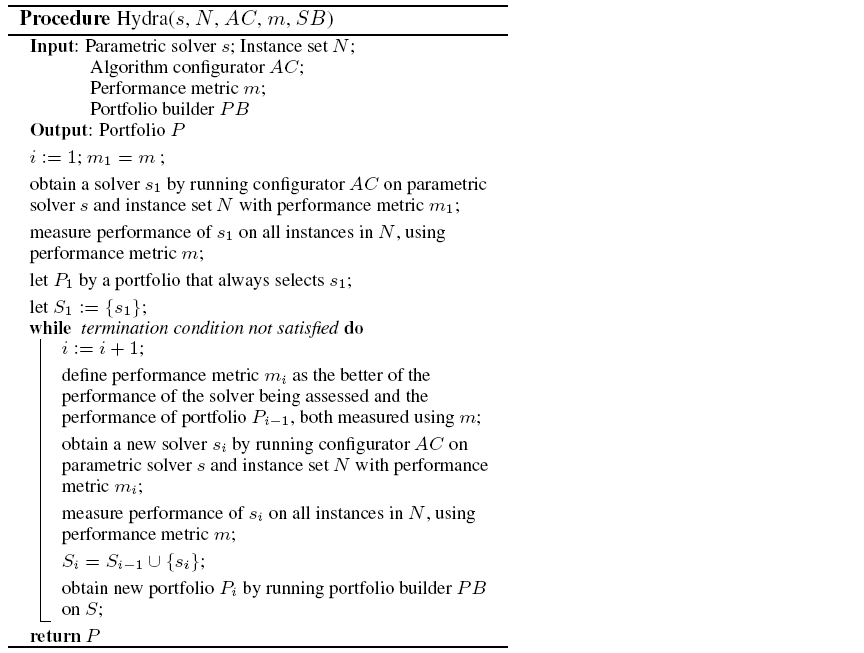## Introduction of Hydra

The AI community has achieved great success in designing high-performance algorithms for hard combinatorial problems, given both considerable domain knowledge and considerable effort by human experts. Two influential methods aim to automate this process: portfolio-based algorithm selection and automated algorithm configuration. The latter has the advantage of requiring virtually no domain knowledge, but produces only a single solver; the former exploits per-instance variation, but requires a set of relatively-uncorrelated candidate solvers. Here, we introduce Hydra : a novel method for combining these two methods, thereby realizing the benefits of both. Hydra automatically builds a set of solvers with complementary strengths by iteratively configuring new algorithms. It is primarily intended for use in problem domains for which an adequate set of candidate solvers does not already exist. Nevertheless, we tested Hydra on a widely-studied domain, stochastic local search algorithms for SAT, in order to characterize its performance against a well-established and highly-competitive baseline. We found that Hydra consistently achieved major improvements over the best existing individual algorithms, and always at least roughly matched?and indeed often exceeded? the performance of the best portfolio of these algorithms.

## How does Hydra work?

The key idea behind Hydra is that a new candidate algorithm should be preferred exactly to the extent that it improves upon the performance of a (slightly idealized) portfolio. Hydra is thus implemented by changing the performance measure given to the algorithm configuration. A candidate algorithm is scored with its actual performance in cases where it is better than the existing portfolio, but with the portfolios in cases where it is worse. Thus an algorithm is not penalized for bad performance on instances for which it should not be selected anyway, but is only rewarded to the extent that it outperforms the portfolio. We note that our method implicitly assumes that a new algorithm will be selected if and only if it would outperform the portfolios previous selection.

As shown in the pseudo-code, Hydra takes five inputs: a parameterized solver $s$, a set of training problem instances $N$, an algorithm configuration procedure $AC$ with a performance metricmto $m$ be optimized, and a procedure for building portfolio-based algorithm selectors $PB$.In its first iteration, Hydra uses configurator $AC$ to produce a configuration of $s$, dubbed $s$1 , that is optimized on training set $N$ according to performance metric $m$. Solver $s$1 is then run on all instances of $N$ in order to collect data that can eventually be input to $PB$; runs performed during the earlier configuration process can be cached and reused as appropriate. We define portfolio $P$1 as the portfolio that always selects $s$1 , and solver set $S$1 as $\left\{s$1} .

Then, in each subsequent iteration $i$, Hydra defines a modified performance metric $m$1 as the better of the performance of the solver being assessed and the performance of the current portfolio, both measured according to $m$. The configurator $AC$ is run to find a configuration $s$i of $s$ that optimizes $m$i on the entire training set $N$. As before, the resulting solver is evaluated on the entire set $N$ and then added to the solver set $S$. We then use $PC$ to construct a new portfolio $P$i from the given set of solvers. We note that in each iteration of Hydra, the size of the candidate solver set $S$i grows by one; however, $PC$ may drop solvers that do not contribute to the performance of portfolio $P$i (this is done, e.g., in SATzilla). Hydra can be terminated using various criteria, such as a user-specified bound on the number of iterations and/or a total computation-time budget.

The algorithm configuration procedure $AC$ used within Hydra must be able to deal efficiently with configurations having equal performance on some or all instances, because such configurations can be expected to be encountered frequently. (For example, all configurations dominated by portfolio $P$i will have equal performance under performance metric $m$i ). It is also possible to exploit $m$i for computational gain when optimizing runtime. Specifically, a run of $s$ on some instance $k$ in $N$ can be terminated during configuration once its runtime reaches portfolio $P$i-1's runtime on $k$.